# Measure of central tendency and Dispersion

Let’s say you have dataset in which one column has numeric data type and there are 1000 data points (rows) in that column, It is hard and time consuming to go through each and every data point, hence to overcome this problem we use descriptive statistics which describes our data and makes our task much more simpler. We also use visualizations such as histogram and boxplot to understand the distribution of the data.

# What is summary statistics?

Rather than understanding 1000 rows, summary statistics only has 1 number which can give the idea of whole data.

There are basically 2 types of summarizing techniques.

1) Measure of central tendency.

• Mean
• Median
• Mode

2) Measure of dispersion.

• Range
• Variance
• Standard deviation
• IQR (Interquartile range)

# Measure of central tendency

1.Mean

2. Median

• Sort the data, return the middle value that would be the median.

3. Mode

• Most frequent value in the data.

# Lets take the example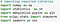importing libraries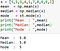Calculate mean, median and mode

Now let’s experiment, we will append extreme values in x and see the changes in mean, median and mode.

• Appending big positive integerAffect of outlier on mean, median and mode
• Appending big negative integer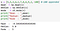Affect of outlier on mean, median and mode

If we add a big positive integer mean followed towards it and if we add a big negative integer mean followed towards it. Notice median and mode remained unchanged.

# Effect of skewness on mean, median and mode.

• Normal distribution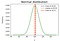Generated using python by author
• Right skewed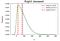Generated using python by author
• Left skewed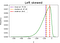Generated using python by author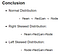Conclusion based on observation from above graphs

# Measure of dispersion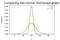Comparing two distribution X1 and X2, we will use them to conclude
• Both data i.e. x1, x2 have the same mean, median and mode however when we visualize the data there is a difference in distribution between x1 and x2.
• X2 which is represented by red has more spread than X1 which is represented in green.

So, for complete understanding of data `Measure of central tendency` alone is not helpful. To understand the data more precisely we need to calculate the measure of spread.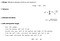Measure of dispersion

# Range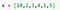Define x

# Variance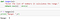Range

# Standard deviation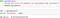Variance

# Interquartile Range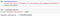Standard Deviation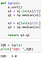Interquartile range

# Concluding on x1 and x2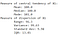Concluding x1Concluding x2

# Conclusion:

• However X1 and X2 have the same mean, median and mode, difference lies in the measure of dispersion.